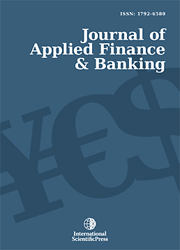# Journal of Applied Finance & Banking

#### Long-Run Relation between Interest Rates and Inflation: Evidence from Turkey

•[ Download ]
• Times downloaded: 7881
• Abstract

The relationship between nominal interest rates and inflation has been frequently explored in both its theoretical and empirical dimensions by many scholars. Most of these studies focus on the influence of inflation on interest rates, while others investigate the effect of interest rates on price levels. The relevant literature begins with the well-known theory of Fisher (1930). According to Fisher’s hypothesis, inflation is the main determinant of interest rates, and as the inflation rate increases by one per cent, the rate of interest increases by the same amount. Following the Fisher Theory, many scholars have sought to examine the interaction between inflation and interest rates. This study examines the relationship between deposit interest rates and the consumer price index in Turkey, employing the threshold vector error correction (T-VEC) analysis. In the first step, a threshold autoregressive (TAR) unit root analysis is applied for the period (2002:01-2011:03) of both variables. Traditional unit root tests are used to test the unit root structure before the cointegration analysis. In the second stage, the Caner and Hansen (2001) TAR unit root test is applied. After determining that the variables are nonstationary, the T-VEC analysis is implemented.ISSN: 1792-6599 (Online)
1792-6580 (Print)